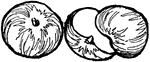### Apples

Two apples. One is whole and the other is split in half.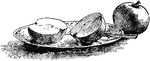### Apples

Two apples. One is cut in half on the plate, beside the knife. The other is still whole.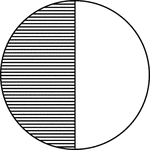### Circle, 1/2 Fraction

Illustration of a circle divided in half.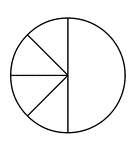### Four Eighths and One Half of a Pie Fraction

A circle subdivided into four eighths and one half.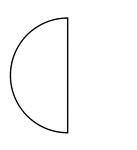### Half of a Fraction Pie

One half of a circle (vertical left).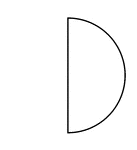### Half of a Fraction Pie

One half of a circle (vertical right).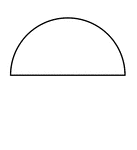### Half of a Fraction Pie

One half of a circle (horizontal top).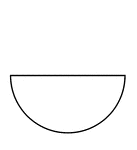### Half of a Fraction Pie

One half of a circle (horizontal bottom).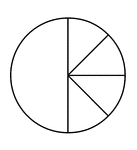### One Half and Four Eighths of a Pie Fraction

A circle subdivided into one half and four eighths.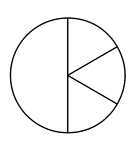### One Half and Three Sixths of a Pie Fraction

A circle subdivided into one half and three sixths.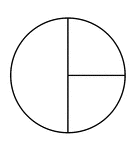### One Half and Two Quarters of a Pie Fraction

A circle subdivided into one half and two quarters.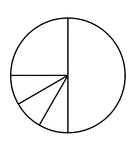### One Quarter, Three Twelfths, and One Half of a Pie Fraction

A circle subdivided into one quarter, three twelfths, and one half.### One Quarter, Two Eighths, and One Half of a Pie Fraction

A circle subdivided into one quarter, two eighths, and one half.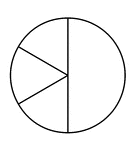### Three Sixths and One Half of a Pie Fraction

A circle subdivided into three sixths and one half.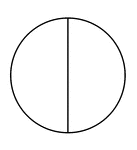### Two Halves of a Fraction Pie

A circle divided in half vertically.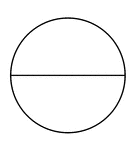### Two Halves of a Fraction Pie

A circle divided in half horizontally.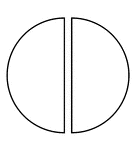### Two Halves of a Fraction Pie

A circle divided in half vertically separated.### Two Halves of a Fraction Pie

A circle divided in half horizontally separated.### Two Quarters and One Half of a Pie Fraction

A circle subdivided into two quarters and one half.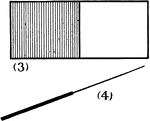### Rectangle, 1/2 Fraction

Illustration of a rectangle divided in half.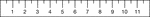### Half Inch Ruler

English ruler with half inch markings.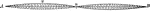### Sound Wave

"Lightly touch the wire at its middle point with the tip of the finger or the beard of a quill; the…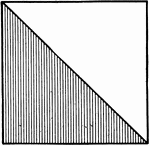### Square, 1/2 Fraction

Illustration of a square divided in half.